# Natural Language Processing in Python

Hey - Nick here! This page is a free excerpt from my new eBook Pragmatic Machine Learning, which teaches you real-world machine learning techniques by guiding you through 9 projects.

Since you're reading my blog, I want to offer you a discount. Click here to buy the book for 70% off now.

Natural language processing - often abbreviated NLP - is the field of machine learning focused on writing code that allows computers to understand natural human language.

NLP combines linguistics, computer programming, and machine learning helps computers read and understand human language.

In this tutorial, you will learn how to build natural language processing models in Python. You'll also learn how to perform text preprocessing in Python, and why preprocessing is such an important part of natural language processing.

## Table of Contents

You can skip to a specific section of Python natural language processing tutorial using the table of contents below:

## Install The Python Natural Language Toolkit

Unlike the other Python packages we have used so far in this course, the Natural Language Toolkit does not come installed by default.

If you installed Python using the Anaconda distribution, you can install the Natural Language Toolkit with the following command in your terminal:

``conda install nltk``

If you installed Python using `pip` or another tool, you can install `nltk` with the following command in your terminal:

``pip install nltk``

With that out of the way, let's continue building our natural language processing model.

## The Identifier and Data Set We Will Use In This Tutorial

This tutorial will use natural language processing to build a spam filter. To do this, we will be using the `stopwords` identifier that is included with the `nltk` library.

To start, open up a Jupyter Notebook. You'll want to first import the `nltk` library into your Jupyter Notebook with the following command:

``import nltk``

Next, you will want to trigger the `nltk` download shell with the following statement:

``nltk.download_shell()``

This will trigger an interactive shell environment that looks like this: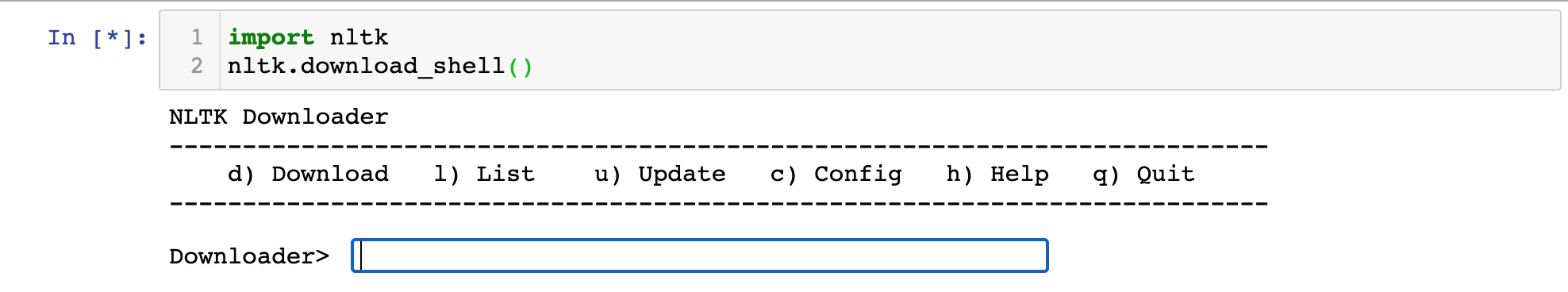In this shell you will need to type `d` then `Enter` to specify that you're downloading a package.

From there, type in the `stopwords` identifier for the corpus that we are trying to download, and hit `Enter` again.

Pressing `q` and `Enter` once this is completed will close the interactive `nltk` shell.

Here is an image of what this should look like when you are done: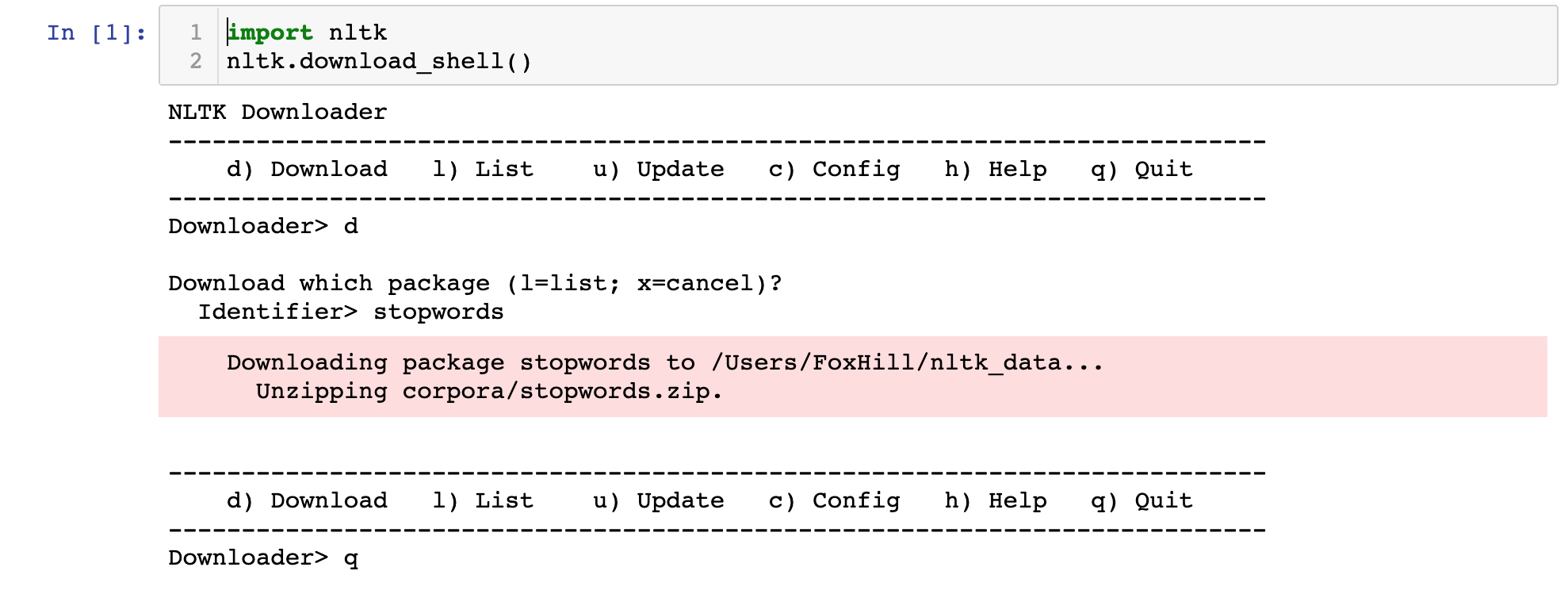Our identifier has been imported. Our next step is to import our data set.

The data set we'll be using is the SMS Spam Collection Data Set made available from the UCI Machine Learning Repository. This data set is a collection of 5,574 SMS messages that have tagged as being either legitimate or spam.

The first step of importing this data set is downloading the file that contains the data. Click here to download it as a zip file. You'll want to click on the zip file after it downloads to decompress the actual file - which is called `SMSSpamCollection`.

Next, move this `SMSSpamCollection` file into the same folder as your Jupyter Notebook.

Lastly, run the following command:

``data = [line.rstrip() for line in open('SMSSpamCollection')]``

This will create a Python list where every item in the list is a different SMS message form the SMS Spam Collection Data Set.

## Examining Our Data Set

We now have a Python list named `data` that contains more than 5000 text messages.

Let's examining the text message with index `10` by using the `data` command. It generates:

``"ham\tI'm gonna be home soon and i don't want to talk about this stuff anymore tonight, k? I've cried enough today."``

This message starts with `ham` to indicate that it is not a spam message. In case you're not familiar, spam and ham and often used as opposites of each other.

Next the message contains `\t`, which implies a tab. The contents of the actual text message round out the remainder of the list item.

These tab separators indicate that our data set is stored inside of a tab-separated value file. This means that it is easy to read the data set into a pandas DataFrame!

Before that, though, we'll need to import various libraries we'll need in our script. Let's handle that next.

## The Libraries You Will Need In This Tutorial

This tutorial will make use of a number of open-source Python libraries, including NumPy and pandas.

Here is a general suite of imports you should run before proceeding through the remainder of this article:

``````import pandas as pd

import numpy as np

import matplotlib.pyplot as plt

import seaborn as sns

%matplotlib inline``````

Now that we've imported our data libraries, we can now reconfigure the SMS Spam Collection Data Set into a pandas DataFrame in our Python script.

You can do this using the following statement:

``data_frame = pd.read_csv('SMSSpamCollection', sep = '\t', names = ['type', 'message'])``

Let's now explore the data set by performing some exploratory data analysis.

## Exploratory Data Analysis

Exploratory data analysis is the process of learning about a data set by calculating summary statistics and creating data visualizations.

It is an important part of any machine learning process.

Let's learn more about the SMS Spam Collection Data Set by using some basic exploratory data analysis techniques.

### Counting the Unique Messages In The Data Set

Running the `describe` method on our pandas DataFrame will generate the following output: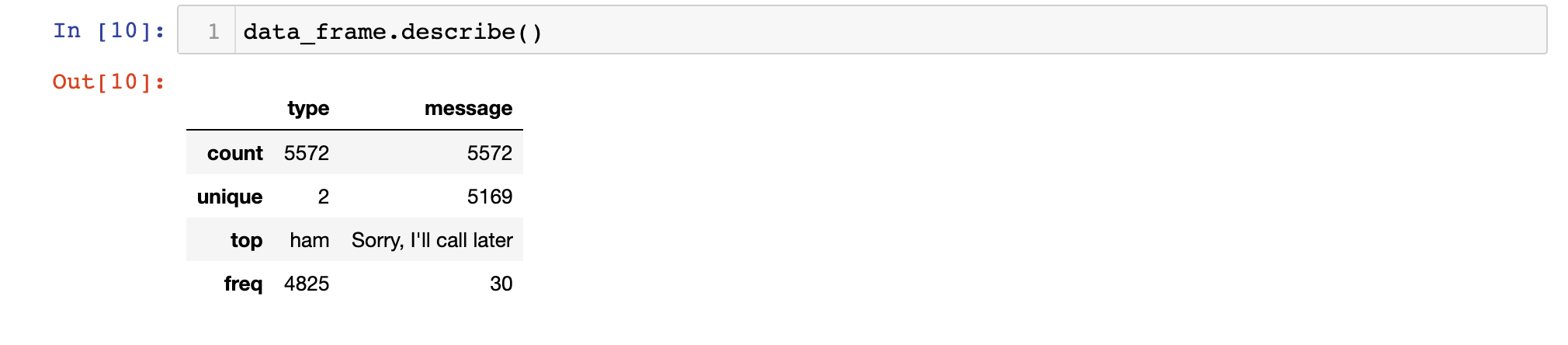The `describe` method is an excellent tool for learning more about a data set.

In this case, the most interesting takeaway from using the describe method is that the number of unique messages in the data set is smaller than the number of total messages in the data set. This implies that there are duplicate messages - likely basic messages like `Yes` and `No`.

### Examining The Differences Between `ham` and `spam` Messages

We can combine the pandas `groupby` method with the `describe` method to get a sense of the differences between the `ham` and `spam` categories. Here's what happens when you do this: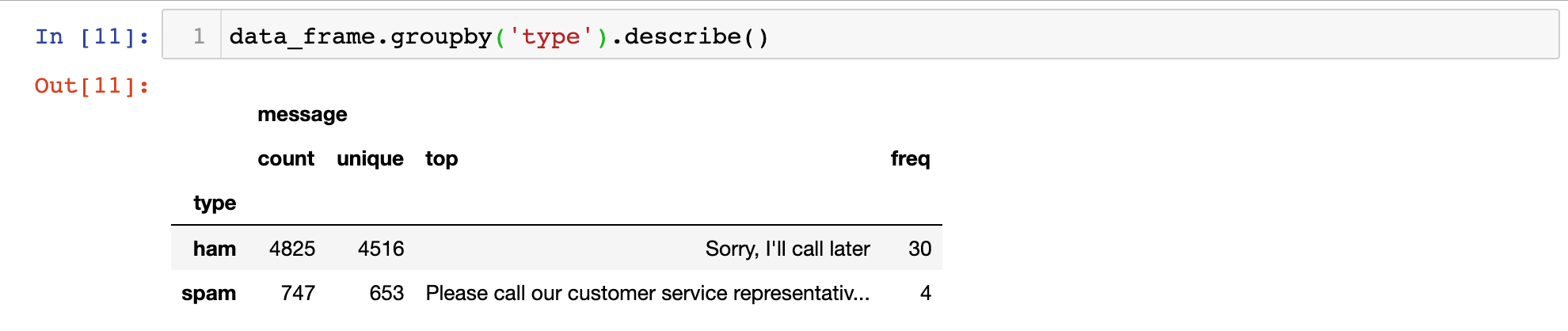As you can see, the most common `ham` message is `Sorry, I'll call later`. The most common `spam` message starts with `Please call our customer service representative`.

We now have a basic sense of the structure of the data within our SMS Spam Collection Data Set. Let's move on to selection the features for our machine learning algorithm in the next section.

### Feature Engineering & Visualizing Message Length

Feature engineering - which is the process of deciding which factors to use in training your model - is an extremely important part of building natural language processing models.

The better you understand your data set, the more likely you are to be equipped to select its best features.

To start, let's add a new column to our DataFrame that contains the length of each text message in the data set:

``data_frame['message length'] = data_frame['message'].apply(len)``

Now that we have data on the length of each message, we can generate a plot of the distribution of message lengths using `seaborn` with the following statement:

``sns.distplot(data_frame['message length'])``

This generates the following data visualization: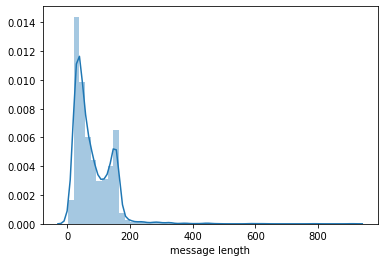This data set seems to be bimodal in nature, which means it has two peaks when presented in a histogram. This might suggest that there are two `average` points - one for `spam` messages and one for `ham` messages!

Let's investigate this by creating separate subplots for both `spam` and `ham` messages:

``data_frame.hist(column='message length', by='type', figsize=(13,5))``

This generates:While this image is far from the most aesthetically appealing visualization we have created in this course, it does show that the two data sets have meaningfully different distributions of message length.

More specifically ,spam messages ten to be longer (they have a higher mean) and ham messages have far more dispersion.

## Text Preprocessing for Natural Language Processing Models

Since the data set we are working with in this tutorial comes in the form of strings, then the classification algorithms that we have used so far in this course (like logistic regression or k-nearest neighbors) cannot be used right away.

We need to perform a process called `text preprocessing` to address this.

### Removing Punctuation

Text preprocessing allows you to transform text into numerical formats. More specifically, we often transform documents in a corpus into a bag of words, just like we did with `black dog` and `brown dog` earlier in this course!

One of the first steps of text preprocessing is to remove punctuation - like `!`, `.`, or `?` - from every document in our corpus.

To do this, we will be relying on Python's `string` library. Import this library into your script with the following statement:

``import string``

This `string` library contains a Python string called `string.punctuation` which lists every character that is considered to be punctuation. Here's the string:

``!"#\$%&\'()*+,-./:;<=>?@[\\]^_`{|}~``

We'll use this `string.punctuation` object shortly to remove these punctuation characters from every document in our corpus.-

### Removing Stop Words

Another important step of text preprocessing is the removal of `stop words`, which are non-meaningful words like `the` or `a`. The `stopwords` identifier that we imported from the `nltk` library earlier in this tutorial will be very useful for this.

We'll need to start our stop words removal process by importing the `stopwords` object from `nltk.corpus` with the following statement:

``from nltk.corpus import stopwords``

This `stopwords` object that we've just imported is a Python class instance. It is not yet an actual list of stopwords.

We must call the `words` method on this object and pass in our desired language to get a list of stopwords, like this:

``stopwords_list = stopwords.words('english')``

This new `stopwords_list` variable is a Python list where each element in the list is a string that is considered to be a stop word.

### Performing Text Preprocessing On A Sample Message

Let's now perform our first two text preprocessing techniques - the removal of punctuation and stop words - on a sample message.

Here's the message we'll be using:

``sample_message = 'This is a sample message! It has punctuation...will we be able to remove it?'``

First, let's remove its punctuation. We'll use Python list comprehension combined with the `join` method to do this.

Here's the full statement:

``message_without_punctuation = ''.join([char for char in sample_message if char not in string.punctuation])``

This new `message_without_punctuation` variable now stores the following value:

``'This is a sample message It has punctuation will we be able to remove it'``

As you can see, we have successfully removed punctuation from our sample message. Let's use a similar technique to remove stop words from the message;

``cleaned_message = ' '.join([word for word in message_without_punctuation.split() if word.lower() not in stopwords_list])``

This `cleaned_message` variable now stores the following string:

``'sample message punctuation able remove'``

This string has been cleaned of both punctuation and stop words.

Let's now formalize all of this logic into a Python function. We can then apply the function to every document in our SMS Spam Collection Data Set.

### Building A Text Preprocessing Function

Let's begin by creating an empty function named `preprocessor` that accepts a string named `message`.

``def preprocessor(message):``

Next let's write a useful docstring that explains the functionality of `preprocessor`:

``````def preprocessor(message):

"""

This function accepts a SMS message and performs two main actions:

1. Removes punctuation from the SMS message

2. Removes stop words (defined by the nltk library) from the SMS message

The function returns a Python list.

"""``````

Now let's add the punctuation and stop words removal functionality that we explored in the last section:

``````def preprocessor(message):

"""

This function accepts a SMS message and performs two main actions:

1. Removes punctuation from the SMS message

2. Removes stop words (defined by the nltk library) from the SMS message

The function returns a Python list.

"""

message_without_punctuation = ''.join([char for char in message if char not in string.punctuation])

return [word for word in message_without_punctuation.split(' ') if word.lower() not in stopwords.words('english')]``````

Now that the function has been defined, let's test the `preprocessor` function on our `sample_message` by running the following statement:

``preprocessor(sample_message)``

This returns:

``['sample', 'message', 'punctuation', 'able', 'remove']``

It looks like our `preprocessor` function is working as intended! We can now move on to applying this function to every document in our corpus.

### Tokenizing Our Data Set

The action of applying a preprocessing algorithm to every document in a corpus is sometimes called `tokenizing`. In this section, we will tokenize the SMS messages inthe SMS Spam Collection Data Set.

To do this, we will use Python's `apply` method. Here's the statement to do this:

``data_frame['message'] = data_frame['message'].apply(preprocessor)``

Note that given the size of the data set and the complexity of this function, this statement may take several seconds to complete in your Jupyter Notebook.

This tokenization step is actually included in the model we'll be using to create our bags of words later on in this tutorial, so you'll want to comment out this step and re-import your data set.

Said differently, this step was presented only to help you better understand what's happening under the hood in this natural language processing machine learning model.

### Vectorizing The Data Set

The next step in our text preprocessing is to `vectorize` the data set, which is a fancy term that simply means transforming each message in the dataset into a bag of words.

We'll do this in three steps:

1. Counting the number of times that each words appears in each method
2. Normalizing the frequency so that more frequent words get lower relative weight (this is called inverse document frequency)
3. Normalize the vectors to their unit length, which will allows us to compare messages with different numbers of characters in them

We will be using `scikit-learn`'s `CountVectorizer` model to do this. This model converts documents within a corpus to a matrix of token counts. Note that since there are so many text messages in our data set and peoples' vocabularies are highly varied, there will be many entries of `0` in the token count matrix.

Let's begin by importing the `CountVectorizer` model:

``from sklearn.feature_extraction.text import CountVectorizer``

Next, let's create an instance of the `CountVectorizer` class and call it `bag_of_words_builder`.

The `CountVectorizer` model takes in a number of arguments, but we can simplify our bag of words creation greatly by just passing in the argument `analyzer = preprocessor`, which tells the model to create the bags of words based on the `preprocessor` function we created earlier.

Lastly, we will call the `fit` method on this model and pass in the pandas Series `data_frame['message']`.

Here is the full statement:

``bag_of_words_builder = CountVectorizer(analyzer = preprocessor).fit(data_frame['message'])``

Let's see how many words are in our bag of words with the following statement:

``len(bag_of_words_builder.vocabulary_)``

This returns `11427`, which is the number of unique words in our corpus.

### Testing Our Bag Of Words Transformation

Let's test our bag of words transformation by applying it to the first message in the data set. To do this, first grab the first message and assign it to a variable called `first_message`:

``first_message = data_frame['message']``

Next, you should call the `transform` method on the `bag_of_words_builder` model and pass in `first_message` within an array, like this:

``first_bag_of_words = bag_of_words_builder.transform([first_message])``

We have successfully created our first bag of words! Printing this object reveals that it looks like this:

`````` (0, 1111)	1

(0, 1484)	1

(0, 2061)	1

(0, 4654)	1

(0, 5218)	1

(0, 5219)	1

(0, 5770)	1

(0, 6218)	1

(0, 6907)	1

(0, 6938)	1

(0, 7556)	1

(0, 7669)	1

(0, 8338)	1

(0, 8919)	1

(0, 10967)	1

(0, 11165)	1``````

Each entry corresponds to a word, and the number beside it shows how many times that word appears in the message. The fact that every entry in this bag of words is `1` shows that there are no duplicated words in this SMS message.

To see which words correspond to each numerical index, call the `bag_of_words_builder.get_feature_names` method and pass in the index you're curious about.

For example, the last word in this bag of words has index `11165`. You can print the corresponding word with the following statement:

``print(bag_of_words_builder.get_feature_names())``

This prints `world`, which is indeed in the SMS message. Nice!

### Building A Bag Of Words Matrix

Let's now apply the `transform` method to every message in our data set to create a matrix that contains a bag of words for every message in the data set.

``bag_of_words_matrix = bag_of_words_builder.transform(data_frame['message'])``

This command is fairly computationally intensive, so it may take some time to run to completion.

### Normalizing the Frequency and Unit Length of the Bag Of Words Matrix

We will now normalize the frequency and unit length of each bag of words within our bag of words matrix using the TD-IDF methods described in our last section.

To do this, we will be relying on the `TfidfTransformer` class available from `scikit-learn`. Let's start by importing it:

``from sklearn.feature_extraction.text import TfidfTransformer``

Now let's create an instance of this class and fit it to our bag of words matrix:

``tfidf_builder = TfidfTransformer().fit(bag_of_words_matrix)``

Now let's see what happens when we use this TF-IDF model to transform the bag of words generated from the first message in our data set.

``first_message_tfidf = tfidf_builder.transform(first_bag_of_words)``

Printing this `first_message_tfidf` variable generates the following output:

``````  (0, 11165)	0.23026685592418913

(0, 10967)	0.19073428545061483

(0, 8919)	0.24704652376837993

(0, 8338)	0.17046869292195632

(0, 7669)	0.26403384065473806

(0, 7556)	0.31253856260694546

(0, 6938)	0.1834692413608692

(0, 6907)	0.15158474664662352

(0, 6218)	0.18915557732842803

(0, 5770)	0.24984711892976424

(0, 5219)	0.26870593862526665

(0, 5218)	0.29835184088197164

(0, 4654)	0.31253856260694546

(0, 2061)	0.24203960256420656

(0, 1484)	0.31253856260694546

(0, 1111)	0.2882862016308418``````

These numbers can be interpreted as the relative weight of each word (represented as its bag of word index) relative to all the documents in the corpus.

### Building our TF-IDF Matrix

Using techniques similar to those used in the last section, we will now calculate TF-IDF values for every message in our bag of words matrix.

Here is the statement to do this:

``tfidf_matrix = tfidf_builder.transform(bag_of_words_matrix)``

After about 2500 words of text preprocessing, we are now ready to finally start training our natural language processing model! This shows the importance of data cleaning compared to the actual machine learning techniques used in the field of natural language processing.

## Training Our Natural Language Processing Model

For reasons that are outside the scope of this tutorial, it makes sense to use a multinomial naive Bayes' Theorem model to train our natural language processing model.

To start, we will want to import this model class from `scikit-learn` with the following command:

``from sklearn.naive_bayes import MultinomialNB``

Now let's create an instance of this model and fit it to our `tfidf_matrix` variable. You will also want to pass in the labels front he original data set, which are stored in the pandas Series `data_frame['type']`:

``spam_detector = MultinomialNB().fit(tfidf_matrix, data_frame['type'])``

## Making Predictions With Our Natural Language Processing Model

Let's now use our natural language processing model to make predictions about whether an SMS message qualifies as `spam` or `ham`. More specifically, let's make a prediction about whether the first message in the data set is `spam` or `ham`.

We can do this by passing in the TF-IDF value for the message into a `predict` method called on the `spam_detector` object we just created, like this:

``spam_detector.predict(first_message_tfidf)``

This returns `ham`, which shows that the message we're examining is not spam.

You may have noticed that so far, we have not split our data set into training data and test data. The reason for this is that I wanted you to have a thorough understanding of natural language processing techniques before adding in this important step.

The rest of this tutorial will focus on how to train and test a formal natural language processing model using training data and test data.

## Splitting Our Data Into Training Data and Test Data

Just like we did in our machine learning tutorial, we will now split our data set into test data and training data.

We'll use `scikit-learn`'s `train_test_split` method to do this. Let's start by importing it:

``from sklearn.model_selection import train_test_split``

Next, we can create our training data and test data with the following statement:

``x_training_data, x_test_data, y_training_data, y_test_data = train_test_split(data_frame['message'], data_frame['type'], test_size = 0.3)``

Now that we have divided our data, we have two choices:

• Perform the same text preprocessing techniques on each of the data splits
• Use `scikit-learn`'s data pipeline feature

We will be using the second choice in this tutorial.

## Building Our Data Pipeline

A data pipeline allows us to perform numerous steps on a data set with very little complexity. This will make more sense as we build our data pipeline in this section.

To start, we will need to import the `Pipeline` class from `scikit-learn`:

``from sklearn.pipeline import Pipeline``

We'll need to create an instance of this `Pipeline` object next. Let's give it the creative name of `pipeline`:

``pipeline = Pipeline()``

This `pipeline` variable will hold our data pipeline, which is a list of tuples where each tuple is a transformation that should be made on the data set. Let's start by adding an empty list inside of the `Pipeline` class declaration:

``pipeline = Pipeline([])``

Now we can add our first tuple.

Each tuple within a data pipeline has two elements:

1. The name of the step within the data pipeline
2. The operation that should be performed on each data point for that step of the pipeline

Our first step will be to use the `CountVectorizer(analyzer=preprocessor)` model on each element. We will call this step `create_bow`, since it's purpose is to create a bag of words. Here's what this tuple looks like:

``('create_bow', CountVectorizer(analyzer=preprocessor))``

And here's what this tuple looks like when nested inside of the data pipeline:

``````pipeline = Pipeline([

('create_bow', CountVectorizer(analyzer=preprocessor))

])``````

Similarly, here's how we can create tuples to calculate our TD-IDF matrix and calculate our predictions using the multinational Bayes' Theorem model:

``````pipeline = Pipeline([

('create_bow', CountVectorizer(analyzer=preprocessor)),

('calculate_tfidf', TfidfTransformer()),

('make_prediction', MultinomialNB())

])``````

Our data pipeline is done!

This data pipeline behaves similarly to the other machine learning models we have explored in this course. We need to train it on our training data then we can use it to make predictions on our test data and verify its performance using a `classification_report` and a `confusion_matrix`.

## Training Our Data Pipeline

Let's train our data pipeline. To do this, call the `fit` method on it and pass in our `x_training_data` and `y_training_data` variables, like this:

``pipeline.fit(x_training_data, y_training_data)``

## Making Predictions With Our Data Pipeline

Making predictions on our test data with our data pipeline is very straightforward.

Call the `predict` method on the `pipeline` object and pass in `x_test_data`:

``predictions = pipeline.predict(x_test_data)``

## Measuring The Performance Of Our Data Pipeline

We'll measure the performance of our data pipeline in a similar way that we measured the performance of our other classification algorithms earlier in this course: by using the `classification_report` and `confusion_matrix` functions.

Let's start by importing both of these functions:

``````from sklearn.metrics import classification_report

from sklearn.metrics import confusion_matrix``````

Now let's generate a `classification_report`:

``classification_report(y_test_data, predictions)``

This generates:

``````             precision    recall  f1-score   support

ham       0.95      1.00      0.98      1436

spam       1.00      0.69      0.82       236

accuracy                           0.96      1672

macro avg       0.98      0.85      0.90      1672

weighted avg       0.96      0.96      0.95      1672``````

As you can tell from this `classification_report`, this model performs very well on the test data. Its precision is high for both

Now let's generate a `confusion_matrix`:

``confusion_matrix(y_test_data, predictions)``

This generates:

``````array([[1436,    0],

[  73,  163]])``````

Again, this matrix shows good performance. Of special importance is the complete lack of any false positives in the test data's predictions.

## The Full Code For This Tutorial

You can view the full code for this tutorial in this GitHub repository. It is also pasted below for your reference:

``````#Import the stopwords identifier

import nltk

nltk.download_shell()

#Import the data set

data = [line.rstrip() for line in open('SMSSpamCollection')]

#Run our data imports

import pandas as pd

import numpy as np

import matplotlib.pyplot as plt

import seaborn as sns

%matplotlib inline

#Create our DataFrame

data_frame = pd.read_csv('SMSSpamCollection', sep = '\t', names = ['type', 'message'])

#Exploratory Data Analysis

data_frame.describe()

data_frame.groupby('type').describe()

data_frame['message length'] = data_frame['message'].apply(len)

sns.distplot(data_frame['message length'])

data_frame.hist(column='message length', by='type', figsize=(13,5))

#Text preprocessing

import string

from nltk.corpus import stopwords

stopwords_list = stopwords.words('english')

#Example of text preprocessing

sample_message = 'This is a sample message! It has punctuation... will we be able to remove it?'

message_without_punctuation = ''.join([char for char in sample_message if char not in string.punctuation])

cleaned_message = ' '.join([word for word in message_without_punctuation.split() if word.lower() not in stopwords_list])

#Building a text preprocessing function

def preprocessor(message):

"""

This function accepts a SMS message and performs two main actions:

1. Removes punctuation from the SMS message

2. Removes stop words (defined by the nltk library) from the SMS message

The function returns a Python list.

"""

message_without_punctuation = ''.join([char for char in message if char not in string.punctuation])

return [word for word in message_without_punctuation.split(' ') if word.lower() not in stopwords.words('english')]

#Testing the function

preprocessor(sample_message)

#Tokenizing the data set

# data_frame['message'] = data_frame['message'].apply(preprocessor)

#Vectorizing the data set

from sklearn.feature_extraction.text import CountVectorizer

bag_of_words_builder = CountVectorizer(analyzer = preprocessor).fit(data_frame['message'])

len(bag_of_words_builder.vocabulary_)

#Testing our bag of words transformation

first_message = data_frame['message']

# print(first_message)

first_bag_of_words = bag_of_words_builder.transform([first_message])

# print(first_bag_of_words)

# print(bag_of_words_builder.get_feature_names())

#Creating a bag of words matrix

bag_of_words_matrix = bag_of_words_builder.transform(data_frame['message'])

#Importing the TD-IDF class

from sklearn.feature_extraction.text import TfidfTransformer

#Calculating a TF-IDF value

tfidf_builder = TfidfTransformer().fit(bag_of_words_matrix)

first_message_tfidf = tfidf_builder.transform(first_bag_of_words)

# print(first_message_tfidf)

#Building the TF-IDF matrix

tfidf_matrix = tfidf_builder.transform(bag_of_words_matrix)

#Import the multinomial naive bayes theorem class

from sklearn.naive_bayes import MultinomialNB

#Training the model

spam_detector = MultinomialNB().fit(tfidf_matrix, data_frame['type'])

#Making predictions

print("THE PREDICTION:" + spam_detector.predict(first_message_tfidf))

#Splitting our data into training data and test data

from sklearn.model_selection import train_test_split

x_training_data, x_test_data, y_training_data, y_test_data = train_test_split(data_frame['message'], data_frame['type'], test_size = 0.3)

#Build our data pipeline

from sklearn.pipeline import Pipeline

pipeline = Pipeline([

('create_bow', CountVectorizer(analyzer=preprocessor)),

('calculate_tfidf', TfidfTransformer()),

('make_prediction', MultinomialNB())

])

#Fit the pipeline and make predictions

pipeline.fit(x_training_data, y_training_data)

predictions = pipeline.predict(x_test_data)

#Measure the performance of our natural language processing algorithm

from sklearn.metrics import classification_report

from sklearn.metrics import confusion_matrix

classification_report(y_test_data, predictions)

confusion_matrix(y_test_data, predictions)``````

## Final Thoughts

In this tutorial, you learned how to build natural language processing models in Python.

Here is a brief summary of what you learned:

• How to install `nltk` on your local machine and import it into your Python script
• How to use the `download_shell()` method to download the `stopwords` identifier
• Learned about the importance of feature engineering and how to use exploratory data analysis to identify possible features (like `message length`) in a data set
• How to perform text preprocessing for a natural language processing model, including the process required to create a bag of words for each message in the SMS Spam Detection Data Set
• How to calculate TD-IDF scores
• How to build a data pipeline and make predictions with it